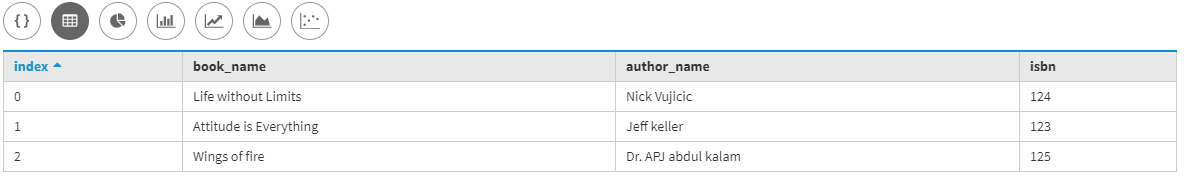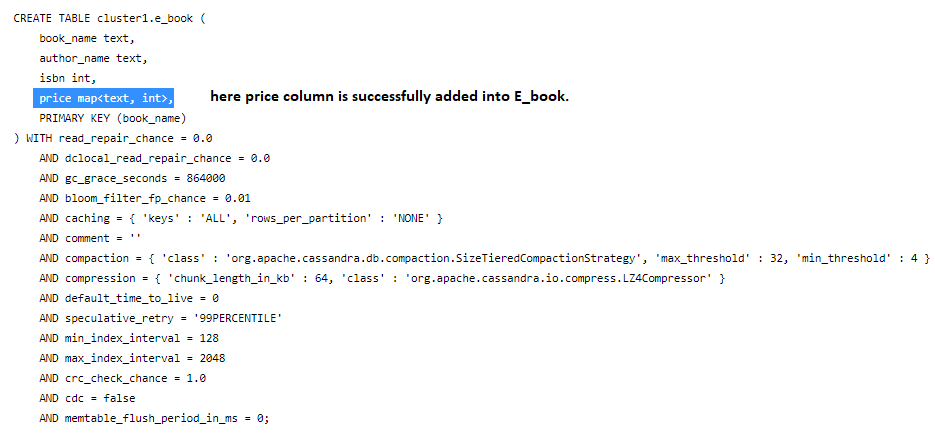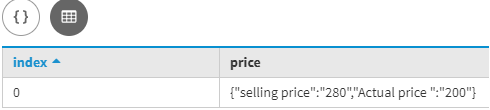# Altering a table to add a collection data type in Cassandra

• Last Updated : 13 Dec, 2019

In this article, we will discuss how we can alter a table to add MAP collection data type and how we insert data after altering the table with the help of the UPDATE command. Let’s discuss one by one.
First, we are going to create a table let’s consider E_book is a table name and Bookk_name, Author_name, Selling_price are the fields in the E_book table. Let’s have a look.

```Create table E_book
(
Book_name text,
Author_name text,
ISBN int,
Primary key(Book_name)
); ```

Now, here we will insert some data by using the following CQL query given below.

```Insert into E_book(Book_name, Author_name, ISBN)
Values ('Attitude is Everything', 'Jeff keller', 123 );

Insert into E_book(Book_name, Author_name, ISBN)
Values ('Life without Limits', 'Nick Vujicic', 124 );

Insert into E_book(Book_name, Author_name, ISBN)
Values ('Wings of fire', 'Dr. APJ abdul kalam', 125 ); ```

Let’s see the results,

```select *
from E_book; ```

Output:Now, here we will add a new collection data type column by using ALTER TABLE command. Let’s have a look.

```ALTER TABLE E_book

Now, to verify used the following cqlsh query.

`describe table E_book; `

Output:In the above Cassandra Query Language (CQL) query, we are using MAP collection in which we insert key pair values. Now, after successfully alter the table we will use the UPDATE command to insert data.

Let’s have a look.

```UPDATE E_book
SET price = price + {'selling price' : 280, 'Actual price ' : 200}
WHERE Book_name = 'Attitude is Everything'; ```

Now, here to verify the result we will use the following CQL query given below.

```SELECT price FROM E_book
WHERE Book_name = 'Attitude is Everything';```

Output:My Personal Notes arrow_drop_up